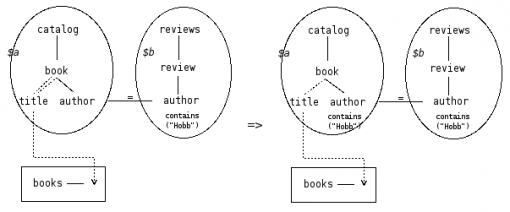To optimize the XQuery evaluation, a search-strategy is needed.
This strategy relies on rule valuation with an coefficient of improvement []. This coefficient is directed by information such as cost model.
We define TGV transformation rules that transforms a given execution plan into an equivalent execution plan (ie. that give the same result when evaluated).
Transformation rules can be logical (ie. based on the algebraic properties of the operators), physical (ie. based on the hardware, system and data statistics, formulas ad coefficients) or user-defined (ie. defined arbitrarily by the user or the administrator according to its knowledge of some particular specificities on datas and sources, or expected results).

## Rule Definition Language

We define the rules definition language.
A rule consists in a rule condition followed by a rule conclusion.
Operations applying on the rule condition to result in the rule conclusion is called a rule transformation.

 FOR [Variables] IF [Conditions] THEN [Modifications]

Table 1: Rule Language

In table 1, we propose a rule definition language which contains three clauses: FOR, IF, THEN. Each one defines a rule property:

• FOR: Entry type which defines search space on rules concerned elements. Type can be: Node, NodeLink, TreePattern, Hyperlink, Constraint.
• IF (for rules conditions): Rule applying conditions on elements defined into the FOR clause. ADT operations are used to define conditions, and temporary variables are used to facilitate manipulations. Each temporary variable will be declared into the form: \$var:= ….
• THEN (for rules conclusion): Transformations to apply if all IF clause operations are verified. We can use variables defined into the previous clause to modify values. Transforming operations are creation, destruction and updates.

## Rule Patterns

Like tgv mappings on XML documents with Tree Patterns, we represent rules in intuitive manners in order to build a pattern to map visually on tgv representations. This rule modelling is called Rule Pattern Model (RP Model).
The RP Model consists in two patterns: condition pattern and conclusion pattern.
Part(s) of the TGV that match the condition pattern must be replaced by the conclusion pattern.1)A logical Rule Pattern

Figure 1 shows a logical rule example: a constraint of one member of a join hyperlink also apply to the other member.2)A Physical Rule Pattern

Figure 2 shows another user-defined rule example: a descendant optional nodelink can be replace by the full path with optional child link.3)Application of the first transformation (figure 1)

Figure 3 illustrates the transformation applied to the TGV on the left sides using the two previous rules. We obtain the TGV on the right side.